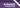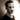More Functional Patterns: Function composition and pointfree styleHey, and welcome back to the third and final part of chapter 7 of Haskell Programming From First Principles! Today we'll be looking at function composition and pointfree style; two related topics that are very commonly seen in Haskell.

## Function composition

Let's start of with function composition. Function composition allows us to create new functions by combining existing ones. The one criterion for composing two functions is that the return value (range) of the first function matches the input value (domain) of the second function. In Haskell, we use the dot operator (`.`) to compose two functions.

Let's inspect the type signature of the `.` operator and see if we can unpack it:

``(.) :: (b -> c) -> (a -> b) -> a -> c``

At first, this may seem a bit complicated, so let's break it down. The `.` operator goes between two functions (like this: `f . g`) and returns a function that goes from `a` to `c`. What it does is to simply 'glue' the two provided functions together.

I quite like Scott Wlaschin's explanation of it from his talk The Power of Composition (this link takes you to the point in the talk that talks about gluing together functions, but I highly recommend checking out the whole thing if you're interested), where he talks about gluing together a transformation from an apple to a banana, and a function from a banana to a cherry to create a function from an apple to a cherry.

The simplest way to explain composition may be to say that you perform an operation on a value, and then pass the result of that operation to the next function. That new function which puts those two together is the composed function. You might see an example like this:

``(f . g) x = f (g x)``

This tells us that applying `f` composed with `g` to `x` is the same as applying `g` to the argument `x` and then applying `f` to the result of that. Note that the `.` operator might initially appear to read backwards: It's the last function to get run that gets written out first. This is because of it's mathematical roots, where people use the '∘' symbol for composing functions (so our `f . g` would be \$f ∘ g\$). I suggest reading the symbol as 'after', which makes it 'f after g', for instance.

If this is all a bit abstract and jargon-y, why don't we look at a concrete example. Say we want a function that tells us whether a string is of even length or not. In that case, we can express the full function as a composition of two smaller functions (the signatures have been simplified/specialized for the sake of example; check the REPL for more): `even :: Int -> Bool`, and `length :: String -> Int` (so it'd be '`even` after `length`'). Notice that the return type of `length` matches the input type of `even`, and that the type of `isOfEvenLength` matches the combination of the functions.

``````isOfEvenLength :: String -> Bool
isOfEvenLength = even . length``````

The astute reader (that's you!) might notice that the function we defined as the composition of the other two functions (`isOfEvenLength`) doesn't list any arguments in its definition. This might look strange but it takes us nicely into our next point: pointfree style.

## Pointfree style

Pointfree style is a way of writing functions without specifying their arguments, most notably when working with composition. It's called 'pointfree' because the arguments are also known as 'points'. There are arguments both for and against writing pointfree style, and it's true that excessive use may cause code to become harder to understand, but used sensibly it can make code tidier, cleaner, and let's us put the focus on the transformations, the functions, rather than the data supplied to them.

Using one of the examples from before, we now get:

``````(f . g) x = f (g x)
-- the above now becomes
f . g = \x -> f (g x)

-- similarly, we can add more functions
f . g . h = \x -> f (g (h x))``````

How does this work? Well, it all goes back to the lambda calculus and currying. Let's take a step back and look at how you could write an alias for a function in Haskell:

``````f a b = -- ... implementation

g = f``````

In the above code, we have defined `g` as simply being the same as `f`. We'll still need to provide it with the same arguments (`a` and `b`), but it's just given a different name. If we want to, we can choose to define it as a specialized version of `f`, with the first argument already applied:

``````f a b = -- ... implementation

g = f 2``````

In this case, `g` is a partially applied version of `f` and is a function from one argument to a result (whatever `f` returns). We could also write the above as `g x = f 2 x`, but because of how partial application works, there's no need to do this. We've already said that `g` is the same as `f` applied to `2`, and that naturally returns a function from one argument to the result.

Similarly, we might do it for something like `map`. Say we want a function that doubles all values in a list:

``````doubleVals = map (*2)
-- is the same as
doubleVals xs = map (*2) xs``````

The two definitions above are equivalent, they're just written out differently.

Whether you prefer pointfree or 'pointful' styles is an individual thing, and is probably based on experience and circumstance. As mentioned earlier, too much and it makes your code hard to read, too little and you're writing out way more than what you need to and taking focus away from the important parts. Like with so many things, try and apply the Goldilocks principle. As always, the Haskell wiki has more detailed information on the topic, so go give that a read if you fancy.

## Definitions

As it's the last post for this chapter, let's go over the chapter definitions.

Anonymous function
Often known as a lambda. It is a function that isn't bound to an identifier and is instead just used as an argument to another function or the like. For instance, `\x -> x` is an anonymous version of the `id` function (`id x = x`).
Currying
The act of transforming a function which takes multiple arguments to a series of nested functions that each take a single argument and return the next function in line (or the result if we're at the end). In Haskell, every function is curried, and this is baked into the language so you don't have to worry about it.
Pattern matching
A way to deconstruct various data types to do something with---or based on---their contents.
Bottom
Bottom is a non-value used to denote that a program cannot return a value or a result. A simple example would be an infinitely looping function, but values that don't handle all their inputs and throw errors also apply here. We'll talk more about Bottom in the chapter on non-strictness.
Higher-order functions
Functions that take other functions as arguments or return functions themselves.
Composition
A way of gluing together multiple functions such that each each function is applied to the result of the next function. Creates a pipeline of transformations.
Pointfree
Also known as 'tacit programming'. Programming without mentioning arguments explicitly.Thomas Heartman is a developer, writer, speaker, and one of those odd people who enjoy lifting heavy things and putting them back down again. Preferably with others. Doing his best to gain and share as much knowledge as possible.